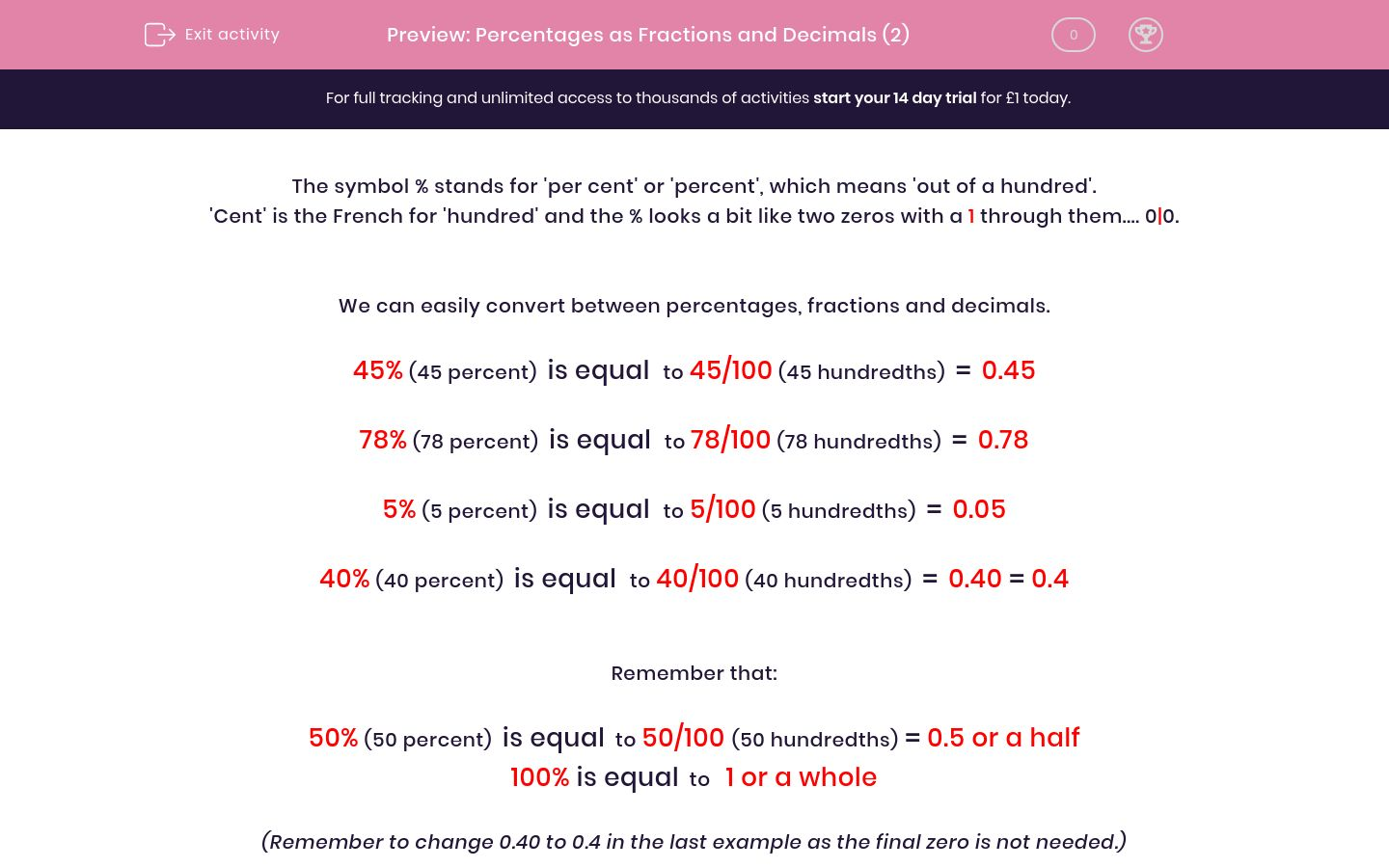# Percentages as Fractions and Decimals (2)

In this worksheet, students recognise the percent symbol (%) and understand that percent relates to ‘number of parts per hundred’, writing percentages as fractions with denominator 100, and as a decimal.Key stage:  KS 2

Curriculum topic:   Maths and Numerical Reasoning

Curriculum subtopic:   Decimals

Difficulty level:#### Worksheet Overview

The symbol % stands for 'per cent' or 'percent', which means 'out of a hundred'.

'Cent' is the French for 'hundred' and the % looks a bit like two zeros with a 1 through them.... 0|0.

We can easily convert between percentages, fractions and decimals.

45% (45 percent)  is equal  to 45/100 (45 hundredths)  =  0.45

78% (78 percent)  is equal  to 78/100 (78 hundredths)  =  0.78

5% (5 percent)  is equal  to 5/100 (5 hundredths)  =  0.05

40% (40 percent)  is equal  to 40/100 (40 hundredths)  =  0.40 = 0.4

Remember that:

50% (50 percent)  is equal  to 50/100 (50 hundredths) = 0.5 or a half

100% is equal  to   1 or a whole

(Remember to change 0.40 to 0.4 in the last example as the final zero is not needed.)

### What is EdPlace?

We're your National Curriculum aligned online education content provider helping each child succeed in English, maths and science from year 1 to GCSE. With an EdPlace account you’ll be able to track and measure progress, helping each child achieve their best. We build confidence and attainment by personalising each child’s learning at a level that suits them.

Get started